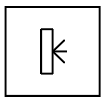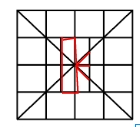Question 15

# Select the option figure in which the given figure is embedded (rotation is NOT allowed).Solution

The following answer figure contains question figure,$$\therefore$$ The correct answer is option D.

• Free SSC Study Material - 18000 Questions
• 230+ SSC previous papers with solutions PDF